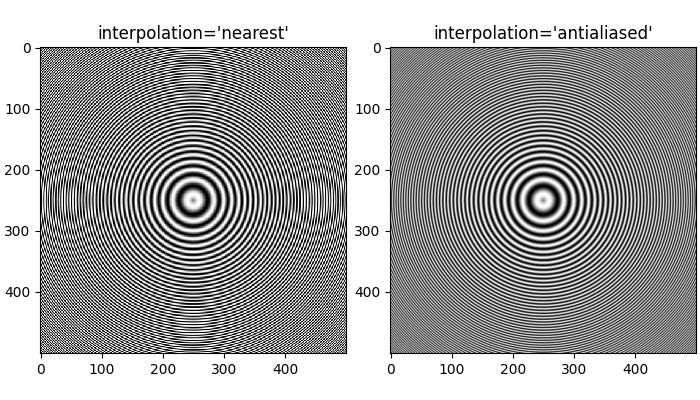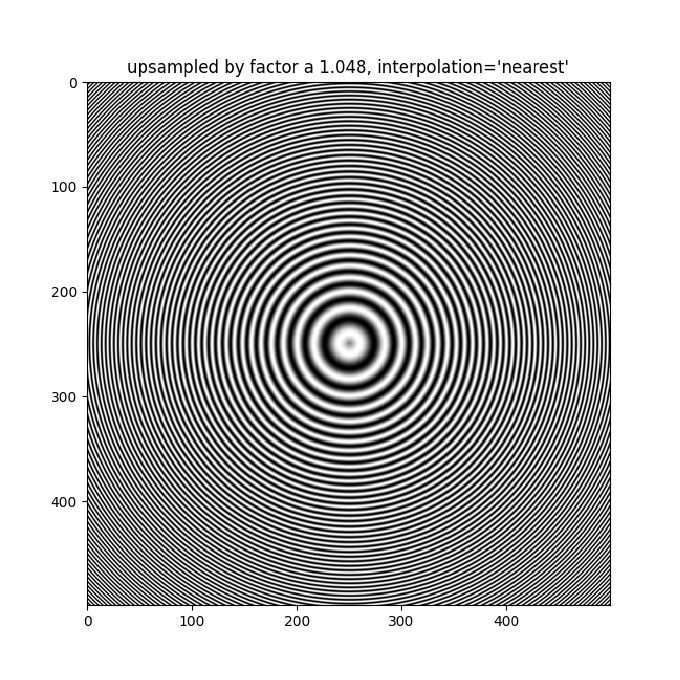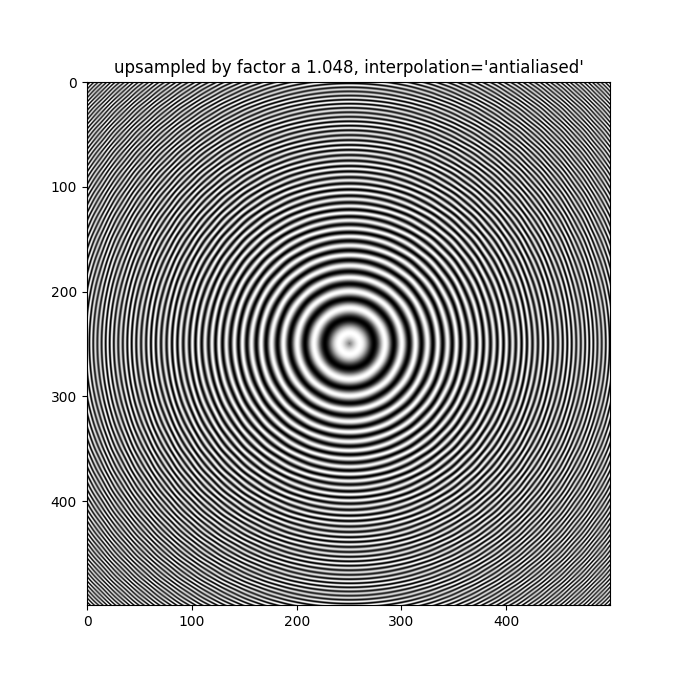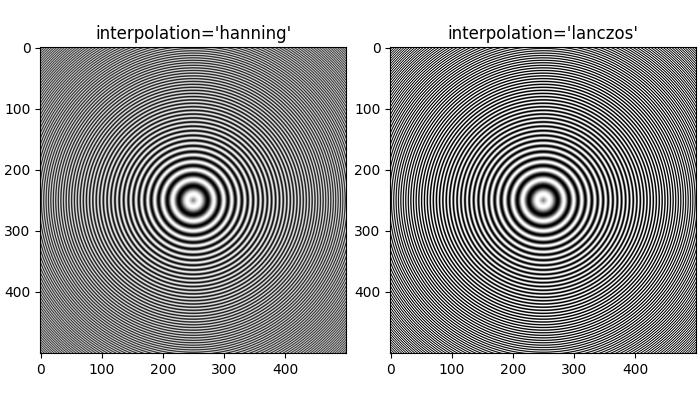Image antialiasing¶

Images are represented by discrete pixels, either on the screen or in an image file. When data that makes up the image has a different resolution than its representation on the screen we will see aliasing effects.

The default image interpolation in Matplotlib is 'antialiased'. This uses a hanning interpolation for reduced aliasing in most situations. Only when there is upsampling by a factor of 1, 2 or >=3 is 'nearest' neighbor interpolation used.

Other anti-aliasing filters can be specified in Axes.imshow using the interpolation keyword argument.

import numpy as np
import matplotlib.pyplot as plt

First we generate a 500x500 px image with varying frequency content:

x = np.arange(500) / 500 - 0.5
y = np.arange(500) / 500 - 0.5

X, Y = np.meshgrid(x, y)
R = np.sqrt(X**2 + Y**2)
f0 = 10
k = 250
a = np.sin(np.pi * 2 * (f0 * R + k * R**2 / 2))

The following images are subsampled from 500 data pixels to 303 rendered pixels. The Moire patterns in the 'nearest' interpolation are caused by the high-frequency data being subsampled. The 'antialiased' image still has some Moire patterns as well, but they are greatly reduced.

fig, axs = plt.subplots(1, 2, figsize=(7, 4), constrained_layout=True)
for ax, interp in zip(axs, ['nearest', 'antialiased']):
ax.imshow(a, interpolation=interp, cmap='gray')
ax.set_title(f"interpolation='{interp}'")
plt.show()Even up-sampling an image with 'nearest' interpolation will lead to Moire patterns when the upsampling factor is not integer. The following image upsamples 500 data pixels to 530 rendered pixels. You may note a grid of 30 line-like artifacts which stem from the 524 - 500 = 24 extra pixels that had to be made up. Since interpolation is 'nearest' they are the same as a neighboring line of pixels and thus stretch the image locally so that it looks distorted.

fig, ax = plt.subplots(figsize=(6.8, 6.8))
ax.imshow(a, interpolation='nearest', cmap='gray')
ax.set_title("upsampled by factor a 1.048, interpolation='nearest'")
plt.show()Better antialiasing algorithms can reduce this effect:

fig, ax = plt.subplots(figsize=(6.8, 6.8))
ax.imshow(a, interpolation='antialiased', cmap='gray')
ax.set_title("upsampled by factor a 1.048, interpolation='antialiased'")
plt.show()Apart from the default 'hanning' antialiasing imshow supports a number of different interpolation algorithms, which may work better or worse depending on the pattern.

fig, axs = plt.subplots(1, 2, figsize=(7, 4), constrained_layout=True)
for ax, interp in zip(axs, ['hanning', 'lanczos']):
ax.imshow(a, interpolation=interp, cmap='gray')
ax.set_title(f"interpolation='{interp}'")
plt.show()